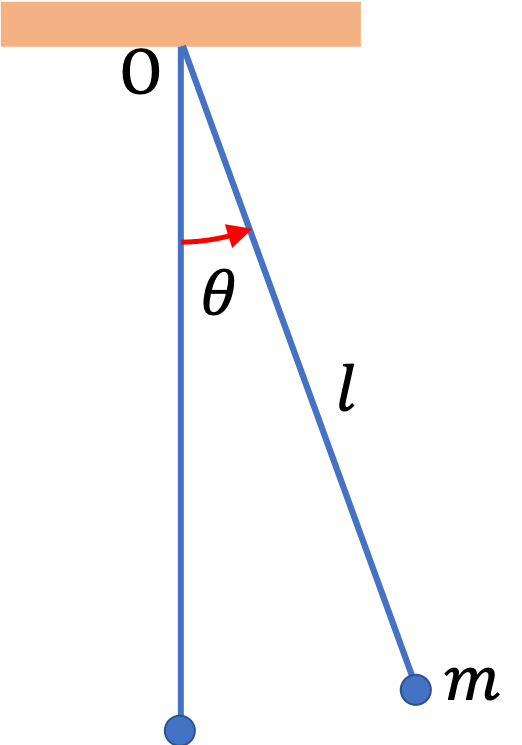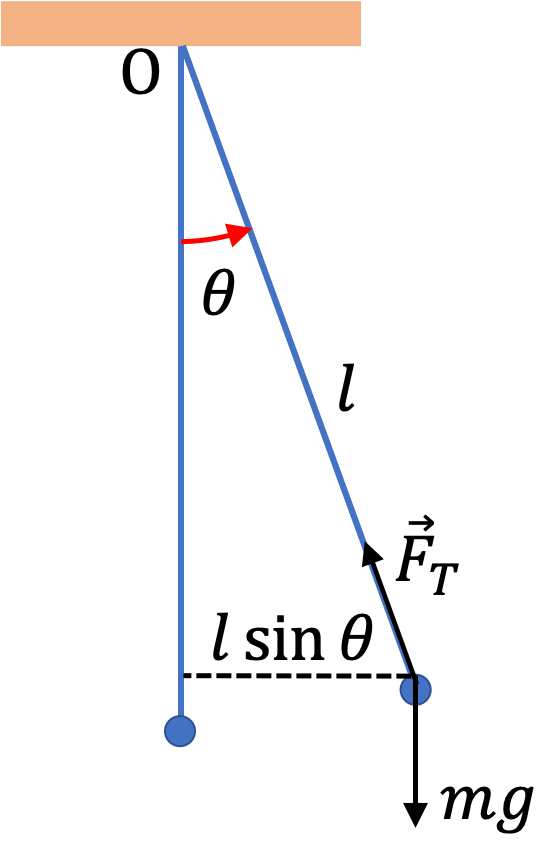## Section13.3Plane Pendulum

A plane pendulum consists of a bob of mass $m$ suspended from a light inextensible cord of length $l$ and swinging in a plane. We will assume that the physical dimension of the bob is much smaller than the length of the cord so that we can treat the bob as a point mass.

A pendulum has a stable equilibrium position when the bob is hanging vertically down from the suspension point. The angular displacement of the bob from the equilibrium is given in terms of angle $\theta$ that the cord makes with the vertical line. The displacement angle is positive for the counter-clockwise change in angle and negative for a clockwise change in angle when viewed from the axis coming out-of-page in the figure.Because we wish to study the angular displacement, it is more convenient to treat the pendulum motion as rotation of the bob about an axis through the point of suspension O perpendicular to the plane of motion. The two forces on the bob are weight $mg$ and tension $F_T\text{.}$ The lever arm of $F_T$ is zero and that of weight is $l\sin\,\theta\text{.}$ Therefore the net torque is

\begin{equation*} \tau_\text{net} = \left( mg l \sin\,\theta, \text{ clockwise sense}\right), \end{equation*}Let $\alpha$ denote the angular acceleration. The moment of inertia of bob about the axis is $I=ml^2\text{.}$ Therefore, the equation of motion is

\begin{equation*} ml^2\alpha = - mg l \sin\,\theta, \end{equation*}

where negative sign is due to the clockwise sense. Simplifying we get

\begin{equation} \alpha = - \dfrac{g}{l}\, \sin\,\theta.\tag{13.3.1} \end{equation}

This equation is not analogous to $a_x =- \omega^2\ x\text{,}$ but if $\sin\theta$ were to be replaced by $\theta\text{,}$ we will get equation where acceleration is prportional to the negative of the displacement. It turns out that for small angles in radians you can replace $\sin\theta$ by $\theta\text{.}$ We call this small-angle approximation.

\begin{equation} \sin\,\theta \approx \theta\ \ \ (\theta\text{ in radians.})\tag{13.3.2} \end{equation}

This approximation is good to two decimal precision for angles $\theta \lt 15^\circ= 0.26\text{ rad}\text{.}$ Therefore, in the small-angle approximation

\begin{equation} \alpha = - \dfrac{g}{l}\, \theta.\tag{13.3.3} \end{equation}

We can use analogy to $a_x =- \omega^2\ x$ to study the motion of the pendulum. Thus, angular frequency of the pendulum will be

\begin{equation} \omega = \sqrt{ \dfrac{g}{l} },\tag{13.3.4} \end{equation}

which means the frequancy of the oscillations

\begin{equation} f = \dfrac{\omega}{2\pi} = \dfrac{1}{2\pi}\,\sqrt{ \dfrac{g}{l} }, \tag{13.3.5} \end{equation}

and the period $T$

\begin{equation} T = \dfrac{1}{f} = 2\pi\,\sqrt{ \dfrac{l}{g} }.\tag{13.3.6} \end{equation}

The formula for the period shows that the time period of a small-amplitude pendulum does not depend on either the mass $m$ of the pendulum bob or the amplitude of oscillations, but only on the length $l$ of the pendulum cord and acceleration due to gravity $g\text{.}$

The dependence of the period on $g$ means that the same pendulum will have different periods on different planets. Also, since the value of $g$ varies over the surface of the Earth, the same pendulum will run at different rates in different locations on the Earth.

Galileo appears to be the first person to notice this aspect of a pendulum motion when he made the observation that different chandeliers of equal length in a church had the same period regardless of their amplitudes of swing or weights. It is said that he timed the swings of chandeliers using his pulses.

(a) A child is swinging on a swing such that her center of mass is $2.0\text{ m}$ from the suspension point. How long does she take to complete one swing?

(b) The child moves to another swing and finds that her swings now take $1.2$ times as much. What is the distance of her center of mass now in this swing?

Hint

(a) Treat swing as a plane pendulum. (b) Use $T \propto \sqrt {L}\text{.}$

(a) $2.84\text{ sec}\text{,}$ (b) $2.88\text{ m}\text{.}$

Solution

(a) Treating the child as a point mass at her center of mass, the swing is just a plane pendulum. Then, the time to complete one swing is the period of the pendulum.

\begin{equation*} T = 2\pi\,\sqrt{ \dfrac{L}{g} } = 2\pi\,\sqrt{ \dfrac{2}{9.81} } = 2.84\text{ sec}. \end{equation*}

(b) Since only length is different, we can use the proportionality, $T \propto \sqrt {L}\text{,}$ to deduce

\begin{equation*} \dfrac{T_2}{T_1} = \sqrt{{L_2}{L_1}}. \end{equation*}

Therefore, $L_2/L_1$ is square of the ratio of times. This gives

\begin{equation*} L_2 = 1.2^2\times 2.0\text{ m}= 2.88\text{ m}. \end{equation*}

Note that length is not $1.2\times\text{.}$ If that was the case, the answer would have been $2.4\text{ m}\text{,}$ but the actual answer is $2.88\text{ m}\text{.}$

The period of a plane pendulum varies from place to place. At place A, the period is $2.84\text{ sec}\text{.}$ At place B, the period is $2.89\text{ sec}\text{.}$ What is the ratio of the acceleration due to gravity, $g_A/g_B\text{?}$

Hint

Use $T\propto 1/\sqrt{g}\text{.}$

$1.05\text{.}$

Solution

Since only thing different may be the acceleration due to gravity, we use the proportionality $T\propto 1/\sqrt{g}$ to obtain

\begin{equation*} \dfrac{T_A}{T_B} = \sqrt{\dfrac{g_B}{g_A}}. \end{equation*}

From this we get

\begin{equation*} \dfrac{g_A}{g_B} = \left( \dfrac{T_B}{T_A} \right)^2 = \left( \dfrac{2.89}{2.82} \right)^2 = 1.05. \end{equation*}

That is, $g$ is about 5% higher at A than at B.